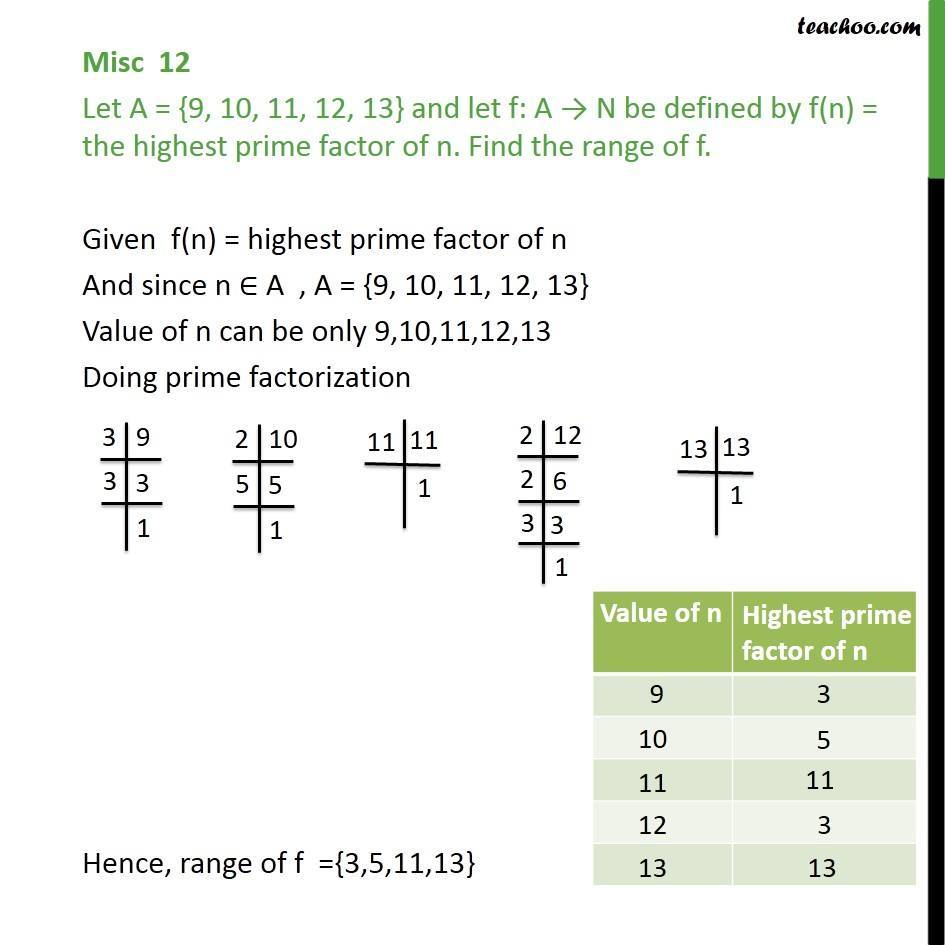1. Chapter 2 Class 11 Relations and Functions
2. Serial order wise
3. Miscellaneous

Transcript

Misc 12 Let A = {9, 10, 11, 12, 13} and let f: A N be defined by f(n) = the highest prime factor of n. Find the range of f. Given f(n) = highest prime factor of n And since n A , A = {9, 10, 11, 12, 13} Value of n can be only 9,10,11,12,13 Doing prime factorization Hence, range of f ={3,5,11,13}

Miscellaneous